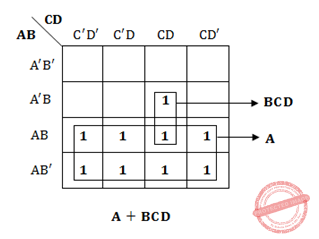# ISRO-2013-28

2.3k views

The most simplified form of the Boolean function

$x (A, B, C, D) = \sum (7, 8, 9, 10, 11, 12, 13, 14, 15)$

(expressed in sum of minterms) is?

1. A + A'BCD
2. AB + CD
3. A + BCD
4. ABC + D
0
i think it should be A also in un simplified form. correct me if i am wrongOption C

selected by
0
option C is correct.Ans: C

1 vote

Option (C)

Following is the solution for the boolean function:option C is correct## Related questions

1
2k views
The binary equivalent of the decimal number $42.75$ is $101010 . 110$ $100110 . 101$ $101010 . 101$ $100110 . 110$
In a three stage counter, using $RS$ flip flops what will be the value of the counter after giving $9$ pulses to its input ? Assume that the value of counter before giving any pulses is $1$ : $1$ $2$ $9$ $10$
How many programmable fuses are required in a PLA which takes $16$ inputs and gives $8$ outputs? It has to use $8$ OR gates and $32$ AND gates. $1032$ $776$ $1284$ $1536$
Two eight bit bytes $1100 0011$ and $0100 1100$ are added. What are the values of the overflow, carry and zero flags respectively, if the arithmetic unit of the CPU uses $2$'s complement form? $0, 1, 1'$ $1, 1, 0$ $1, 0, 1$ $0, 1, 0$